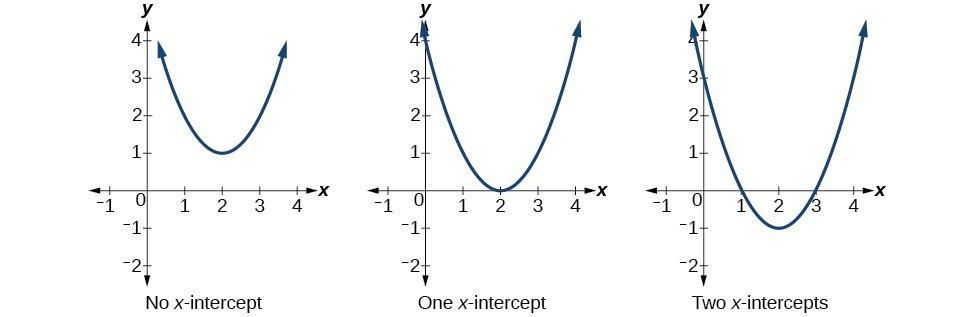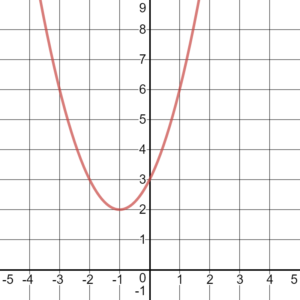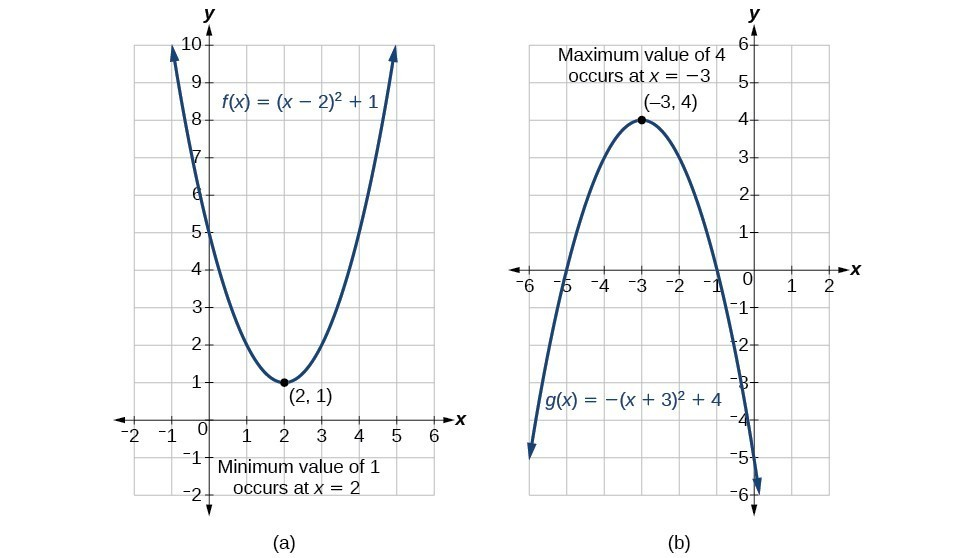### Learning Outcomes

• Use the quadratic formula and factoring to find both real and complex roots ($x$-intercepts) of quadratic functions.
• Use algebra to find the $y$-intercepts of a quadratic function.
• Solve problems involving the roots and intercepts of a quadratic function.
• Use the discriminant to determine the nature (real or complex) and quantity of solutions to quadratic equations.
• Determine a quadratic function’s minimum or maximum value.
• Solve problems involving a quadratic function’s minimum or maximum value.

In this section we will investigate quadratic functions further, including solving problems involving area and projectile motion. Working with quadratic functions can be less complex than working with higher degree polynomial functions, so they provide a good opportunity for a detailed study of function behavior.

Much as we did in the application problems above, we also need to find intercepts of quadratic equations for graphing parabolas. Recall that we find the $y$-intercept of a quadratic by evaluating the function at an input of zero, and we find the $x$-intercepts at locations where the output is zero. Notice that the number of $x$-intercepts can vary depending upon the location of the graph.Number of $x$-intercepts of a parabola

Mathematicians also define $x$-intercepts as roots of the quadratic function.

### How To: Given a quadratic function $f\left(x\right)$, find the Y– and X-intercepts.

1. Evaluate $f\left(0\right)$ to find the $y$-intercept.
2. Solve the quadratic equation $f\left(x\right)=0$ to find the $x$-intercepts.

### Example: Finding the y– and x-Intercepts of a Parabola

Find the $y$– and $x$-intercepts of the quadratic $f\left(x\right)=3{x}^{2}+5x - 2$.

In the above example the quadratic was easily solved by factoring. However, there are many quadratics that cannot be factored. We can solve these quadratics by first rewriting them in standard form.

### How To: Given a quadratic function, find the x-intercepts by rewriting in standard form.

1. Substitute $a$ and $b$ into $h=-\dfrac{b}{2a}$.
2. Substitute $x=h$ into the general form of the quadratic function to find $k$.
3. Rewrite the quadratic in standard form using $h$ and $k$.
4. Solve for when the output of the function will be zero to find the $x$intercepts.

### Example: Finding the Roots of a Parabola

Find the $x$-intercepts of the quadratic function $f\left(x\right)=2{x}^{2}+4x - 4$.

### Try It

Solve ${x}^{2}+x+2=0$.

### Example: Applying the Vertex and x-Intercepts of a Parabola

A ball is thrown upward from the top of a 40 foot high building at a speed of 80 feet per second. The ball’s height above ground can be modeled by the equation $H\left(t\right)=-16{t}^{2}+80t+40$.

a. When does the ball reach the maximum height?

b. What is the maximum height of the ball?

c. When does the ball hit the ground?

### Try It

A rock is thrown upward from the top of a 112-foot high cliff overlooking the ocean at a speed of 96 feet per second. The rock’s height above ocean can be modeled by the equation $H\left(t\right)=-16{t}^{2}+96t+112$.

a. When does the rock reach the maximum height?

b. What is the maximum height of the rock?

c. When does the rock hit the ocean?

## Complex Roots

Now you will hopefully begin to understand why we introduced complex numbers at the beginning of this module. Consider the following function: $f(x)=x^2+2x+3$, and it’s graph below:Does this function have roots? It’s probably obvious that this function does not cross the $x$-axis, therefore it doesn’t have any $x$-intercepts. Recall that the $x$-intercepts of a function are found by setting the function equal to zero:

$x^2+2x+3=0$

In the next example we will solve this equation. You will see that there are roots, but they are not $x$-intercepts because the function does not contain $(x,y)$ pairs that are on the $x$-axis.  We call these complex roots.

By setting the function equal to zero and using the quadratic formula to solve, you will see that the roots are complex numbers.

### Example

Find the $x$-intercepts of the quadratic function. $f(x)=x^2+2x+3$

### Try It

The following video gives another example of how to use the quadratic formula to find complex solutions to a quadratic equation.

## The Discriminant

The quadratic formula not only generates the solutions to a quadratic equation, it tells us about the nature of the solutions. When we consider the discriminant, or the expression under the radical, ${b}^{2}-4ac$, it tells us whether the solutions are real numbers or complex numbers, and how many solutions of each type to expect. In turn, we can then determine whether a quadratic function has real or complex roots. The table below relates the value of the discriminant to the solutions of a quadratic equation.

Value of Discriminant Results
${b}^{2}-4ac=0$ One repeated rational solution
${b}^{2}-4ac>0$, perfect square Two rational solutions
${b}^{2}-4ac>0$, not a perfect square Two irrational solutions
${b}^{2}-4ac<0$ Two complex solutions

### A General Note: The Discriminant

For $a{x}^{2}+bx+c=0$, where $a$, $b$, and $c$ are real numbers, the discriminant is the expression under the radical in the quadratic formula: ${b}^{2}-4ac$. It tells us whether the solutions are real numbers or complex numbers and how many solutions of each type to expect.

### Example

Use the discriminant to find the nature of the solutions to the following quadratic equations:

1. ${x}^{2}+4x+4=0$
2. $8{x}^{2}+14x+3=0$
3. $3{x}^{2}-5x - 2=0$
4. $3{x}^{2}-10x+15=0$

We have seen that a quadratic equation may have two real solutions, one real solution, or two complex solutions.

Let’s summarize how the discriminant affects the evaluation of $\sqrt{{{b}^{2}}-4ac}$, and how it helps to determine the solution set.

• If $b^{2}-4ac>0$, then the number underneath the radical will be a positive value. You can always find the square root of a positive, so evaluating the Quadratic Formula will result in two real solutions (one by adding the square root, and one by subtracting it).
• If $b^{2}-4ac=0$, then you will be taking the square root of 0, which is 0. Since adding and subtracting 0 both give the same result, the “$\pm$” portion of the formula doesn’t matter. There will be one real repeated solution.
• If $b^{2}-4ac<0$, then the number underneath the radical will be a negative value. Since you cannot find the square root of a negative number using real numbers, there are no real solutions. However, you can use imaginary numbers. You will then have two complex solutions, one by adding the imaginary square root and one by subtracting it.

### Example

Use the discriminant to determine how many and what kind of solutions the quadratic equation $x^{2}-4x+10=0$ has.

### Try It

There are many real-world scenarios that involve finding the maximum or minimum value of a quadratic function, such as applications involving area and revenue.### Example: Finding the Maximum Value of a Quadratic Function

A backyard farmer wants to enclose a rectangular space for a new garden within her fenced backyard. She has purchased 80 feet of wire fencing to enclose three sides, and she will use a section of the backyard fence as the fourth side.

1. Find a formula for the area enclosed by the fence if the sides of fencing perpendicular to the existing fence have length $L$.
2. What dimensions should she make her garden to maximize the enclosed area?

The problem we solved above is called a constrained optimization problem. We can optimize our desired outcome given a constraint, which in this case was a limited amount of fencing materials. Try it yourself in the next problem.

### How To: Given an application involving revenue, use a quadratic equation to find the maximum.

1. Write a quadratic equation for revenue.
2. Find the vertex of the quadratic equation.
3. Determine the $y$-value of the vertex.

### Example: Finding Maximum Revenue

The unit price of an item affects its supply and demand. That is, if the unit price goes up, the demand for the item will usually decrease. For example, a local newspaper currently has 84,000 subscribers at a quarterly charge of $30. Market research has suggested that if the owners raise the price to$32, they would lose 5,000 subscribers. Assuming that subscriptions are linearly related to the price, what price should the newspaper charge for a quarterly subscription to maximize their revenue?

In the example above, we knew the number of subscribers to a newspaper and used that information to find the optimal price for each subscription. What if the price of subscriptions is affected by competition?

### try it

Previously, we found a quadratic function that modeled revenue as a function of price.

$\text{Revenue}-2,500{p}^{2}+159,000p$

We found that selling the paper at $\31.80$ per subscription would maximize revenue.  What if your closest competitor sells their paper for $\25.00$ per subscription? What is the maximum revenue you can make you sell your paper for the same?

## Key Equations

The quadratic formula $x=\frac{-b\pm \sqrt{{b}^{2}-4ac}}{2a}$

The discriminant is defined as $b^2-4ac$

## Key Concepts

• The zeros, or $x$-intercepts, are the points at which the parabola crosses the $x$-axis. The $y$-intercept is the point at which the parabola crosses the $y$axis.
• The vertex can be found from an equation representing a quadratic function.
• A quadratic function’s minimum or maximum value is given by the $y$-value of the vertex.
• The minium or maximum value of a quadratic function can be used to determine the range of the function and to solve many kinds of real-world problems, including problems involving area and revenue.
• Some quadratic equations must be solved by using the quadratic formula.
• The vertex and the intercepts can be identified and interpreted to solve real-world problems.
• Some quadratic functions have complex roots.

## Glossary

discriminant
the value under the radical in the quadratic formula, $b^2-4ac$, which tells whether the quadratic has real or complex roots
vertex
the point at which a parabola changes direction, corresponding to the minimum or maximum value of the quadratic function
vertex form of a quadratic function
another name for the standard form of a quadratic function
zeros
in a given function, the values of $x$ at which $y=0$, also called roots